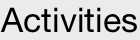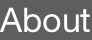ActivitiesTsuyoshi Okubo

University of Tokyo, Japan

13 July 2023 Thu 4.30 pmIBS Center for Theoretical Physics of Complex Systems (PCS), Administrative Office (B349), Theory Wing, 3rd floor

Expo-ro 55, Yuseong-gu, Daejeon, South Korea, 34126 Tel: +82-42-878-8633

To represent a quantum many-body state, we need to treat huge vectors in exponentially increasing dimensions as we increase the number of particles. Such an exponentially large vector space is a fundamental difficulty in treating quantum many-body problems in classical computers. However, when we use a well-controlled quantum system, such as a quantum computer, we may solve quantum many-body problems with a cost polynomial of the number of particles. In recent years, to use a noisy quantum computer for quantum many-body problems, the variational quantum eigensolver (VQE)  has attracted much interest. In the VQE approach, a quantum many-body state is represented as a quantum circuit, and we optimize circuit parameters to minimize the energy expectation value. However, it is still unclear whether the VQE approach has an advantage over classical computation on practical quantum many-body problems.

In this talk, We will discuss the possibility of using tensor network representations of quantum many-body states to design an efficient quantum circuit suitable for near-future noisy quantum computers. As a concrete example, we will consider the spin liquid state in the honeycomb lattice Kitaev model . For this model, a simple tensor network state can capture the qualitative properties of the spin liquid. By adding short-range excitations, we can also systematically improve its energy expectation value . We will show that a similar procedure can be applied to the VQE approach by representing tensor network states as quantum circuits. We will discuss that we can efficiently optimize the infinite system by solving an optimization problem in small clusters through this approach.

 A. Peruzzo, J. McClean, P. Shadbolt, et al., Nat. Commun. 5, 4213 (2014).

 A. Kitaev, Ann. Phys. 321, 2 (2006).

 H.-Y. Lee, R. Kaneko, T. Okubo, and N. Kawashima, Phys. Rev. Lett. 123, 087203 (2019).

1. quantum-classical entangled approach with tensor networks for spin liquid